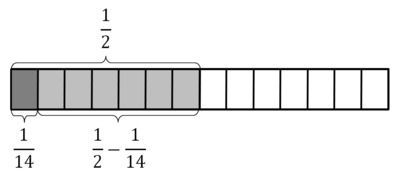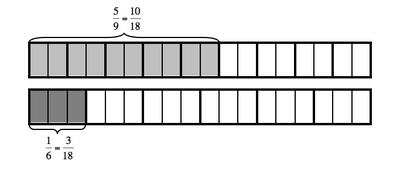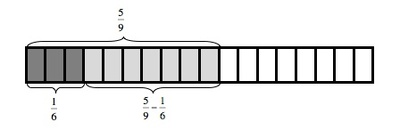# Finding Common Denominators to Subtract

Alignments to Content Standards: 5.NF.A.1

1. To subtract fractions, we usually first find a common denominator.
1. Find two different common denominators for $\frac{1}{2}$ and $\frac{1}{14}$
2. Use each common denominator to find the value of $\frac{1}{2}-\frac{1}{14}$. Draw a picture that shows your solution.
2. Find $\frac{5}{9}-\frac{1}{6}$. Draw a picture that shows your solution.
3. Find $\frac{21}{10}-\frac{24}{15}$.

## IM Commentary

Part (a) of this task asks students to use two different denominators to subtract fractions. The purpose of this is to help students realize that any common denominator will work, not just the least common denominator. Part (b) does not ask students to do it in more than one way; the purpose is to give them an opportunity to choose a denominator and possibly compare with another student who chose a different denominator. The two likely choices are the least common denominator and the product of the two denominators.

Parts (a) and (b) ask students to draw pictures to help them see why finding a common denominator is important. Part (c) does not; the purpose of part (c) is to help students move away from a reliance on drawing pictures. Students can draw a picture if they want, but this subtraction problem is easier to do symbolically, which helps students appreciate the power of symbolic notation.

The numbers were chosen in each part for a specific reason. Part (a) asks students to subtract unit fractions. This helps emphasize the need for a common denominator. Furthermore, one denominator is a multiple of the other, so students should recognize it as a common denominator for both fractions. Part (b) asks students to subtract a unit fraction from a non-unit fraction. Part (c) asks students to subtract a fraction with a larger numerator from a fraction with a smaller numerator. The purpose here is to be sure that students are not simply subtracting numerators and then subtracting denominators, since a student who does that will run into problems right away.

## Solution

1. The denominators of these fractions are 2 and 14. Since 2 divides evenly into 14, 14 is a multiple of 2, so 14 itself is a common denominator for the fractions $\frac{1}{2}$ and $\frac{1}{14}$. The picture below shows this:Here is a picture showing the fractions when they are both written in terms of fourteenths:Any multiple of 14 is a common multiple of 2 and 14. So $2\times14=28$ is common multiple and is a common denominator for the fractions $\frac{1}{2}$ and $\frac{1}{14}$. Thus, 14 and 28 are two different common denominators for the fractions $\frac{1}{2}$ and $\frac{1}{14}$.

2. The first common denominator that we identified in part (i) was 14. Here is a picture that represents $\frac12 - \frac{1}{14}$:This is how we can write the process of finding a common denominator and subtracting using symbols:

\begin{align} \frac{1}{2}-\frac{1}{14} &=\frac{1\times7}{2\times7}-\frac{1}{14}\\ &=\frac{7}{14}-\frac{1}{14}\\ &=\frac{7-1}{14}\\ &=\frac{6}{14} \end{align}
The picture shows that once we have converted $\frac12$ to $\frac{7}{14}$ we can subtract one of the fourteenths and we are left with 6 fourteenths, as we found symbolically.

The second common denominator that we identified in part (i) was 28. This is how we might use this common denominator to solve the given subtraction problem:

\begin{align} \frac{1}{2}-\frac{1}{14}&=\frac{1\times14}{2\times14}-\frac{1\times2}{14\times2}\\ &=\frac{14}{28}-\frac{2}{28}\\ &=\frac{14-2}{28}\\ &=\frac{12}{28} \end{align}Note that \begin{align} \frac{6}{14}&=\frac{3\times 2}{7\times 2}\\ &=\frac{3}{7}\\ &=\frac{3\times 4}{7\times 4}\\ &=\frac{12}{28} \end{align} So we get the same answer using different denominators, as we would expect!

1. In order to find a solution to this subtraction problem, we must first find a common denominator for the fractions $\frac{5}{9}$ and $\frac{1}{6}$. 18 is a common multiple of the denominators 9 and 6 because $9\times2=18$ and $6\times3=18$. This means that 18 is a common denominator for the fractions $\frac{5}{9}$ and $\frac{1}{6}$. Here is a picture that shows both of these fractions when they are written in terms of eighteenths:Here is a picture that represents $\frac{5}{9}-\frac{1}{6}$ using this common denominator:Any other common multiple of the denominators 9 and 6 could also be used when solving this subtraction problem. This is how we write the process of finding the common denominator and subtracting using symbols:

\begin{align} \frac{5}{9}-\frac{1}{6}&=\frac{5\times2}{9\times2}-\frac{1\times3}{6\times3}\\ &=\frac{10}{18}-\frac{3}{18}\\ &=\frac{10-3}{18}\\ &=\frac{7}{18} \end{align}

The picture shows that after we convert both fractions to eighteenths we can subtract 3 eighteenths from 10 eighteenths and are left with 7 eighteenths, the same answer that we found symbolically.

2. In order to find a solution to this subtraction problem, we must again first find a common denominator for the fractions $\frac{21}{10}$ and $\frac{24}{15}$. 30 is a common multiple of the denominators 10 and 15 because $10\times3=30$ and $15\times2=30$. This means that 30 is a common denominator for the fractions $\frac{21}{10}$ and $\frac{24}{15}$. This is how we might use the common denominator 30 in order to solve this subtraction problem using symbols:

\begin{align} \frac{21}{10}-\frac{24}{15}&=\frac{21\times3}{10\times3}-\frac{24\times2}{15\times2}\\ &=\frac{63}{30}-\frac{48}{30}\\ &=\frac{63-48}{30}\\ &=\frac{15}{30} \end{align}
If we pause for a moment, we will see that $\frac{15}{30}$ is equivalent to $\frac{1}{2}$, although either representation of the difference is correct.